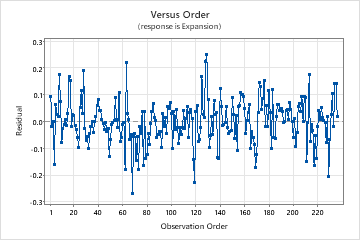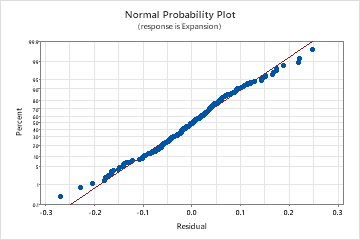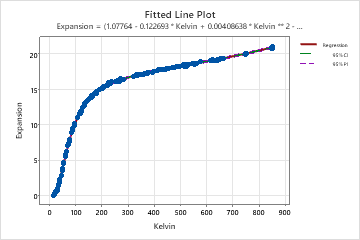# Interpret the key results for Nonlinear Regression

Complete the following steps to interpret a nonlinear regression model. Key output includes the fitted line plot, the standard error of the regression, and the residual plots.

## Step 1: Determine whether the regression line fits your data

If your nonlinear model contains one predictor, Minitab displays the fitted line plot to show the relationship between the response and predictor data. The plot includes the regression line, which represents the regression equation. You can also choose to display the 95% confidence and prediction intervals on the plot.

Evaluate how well the model fits your data and whether the model meets your goals. Examine the fitted line plot to determine whether the following criteria are met:
• The sample contains an adequate number of observations throughout the entire range of all the predictor values.
• The model properly fits the curvature in the data. To determine which model is best, examine the plot, the standard error of the regression (S), and the Lack-of-Fit test when your data contain replicates.
• Look for any outliers, which can have a strong effect on the results. Try to identify the cause of any outliers. Correct any data entry or measurement errors. Consider removing data values that are associated with abnormal, one-time events (special causes). Then, repeat the analysis. For more information on detecting outliers, go to Unusual observations.
On this fitted line plot, the regression line follows the curvature in the points very closely. There does not appear to be any systematic deviations from the fitted line. The points adequately cover the entire range of predictor values.## Step 2: Examine the relationship between the predictors and the response

Use the regression equation to describe the relationship between the response and the terms in the model. The regression equation is an algebraic representation of the regression line. Enter the value of each predictor into the equation to calculate the mean response value. Unlike linear regression, a nonlinear regression equation can take many forms.

For nonlinear equations, determining the effect that each predictor has on the response can be less intuitive than it is for linear equations. Unlike the parameter estimates in linear models, there is no consistent interpretation for the parameter estimates in nonlinear models. The correct interpretation for each parameter depends on the expectation function and the parameter's place in it. If your nonlinear model contains only one predictor, assess the fitted line plot to see the relationship between the predictor and response.

If you need to determine whether a parameter estimate is statistically significant, use the confidence intervals for the parameters. The parameter is statistically significant if the range excludes the null hypothesis value. Minitab cannot calculate p-values for parameters in nonlinear regression. For linear regression, the null hypothesis value for every parameter is zero, for no effect, and the p-value is based on this value. However, in nonlinear regression, the correct null hypothesis value for each parameter depends on the expectation function and the parameter's place in it.

For some data sets, expectation functions, and confidence levels, it is possible that one or both confidence bounds may not exist. Minitab indicates missing results with an asterisk. If the confidence interval has a missing bound, a lower confidence level might produce a two-sided interval.

Convergence on a solution does not necessarily guarantee that the model fit is optimal or that the sum of squared errors (SSE) are minimized. Convergence on incorrect parameter values can occur due to a local SSE minimum or an incorrect expectation function. Therefore, it is crucial to examine the parameter values, fitted line plot, and residual plots, to determine if the model fit and parameter values are reasonable.

## Step 3: Determine how well the model fits your data

To determine how well the model fits your data, examine the statistics in the Model Summary table and the Lack of Fit table.

S

Use S to assess how well the model describes the response.

S is measured in the units of the response variable and represents how far the data values fall from the fitted values. The lower the value of S, the better the model describes the response. However, a low S value by itself does not indicate that the model meets the model assumptions. You should check the residual plots to verify the assumptions.

Lack of Fit

Minitab automatically displays the Lack of Fit table when your data contain replicates. Replicates are multiple observations with identical predictor values. If your data do not contain replicates, it is impossible to calculate the pure error that is required to perform this test. Different response values for replicates represent pure error because only random variation can cause differences between the observed response values.

To determine whether the model correctly specifies the relationship between the response and the predictors, compare the p-value for the lack-of-fit test to your significance level to assess the null hypothesis. The null hypothesis for the lack-of-fit test is that the model correctly specifies the relationship between the response and the predictors. Usually, a significance level (denoted as alpha or α) of 0.05 works well. A significance level of 0.05 indicates a 5% risk of concluding that the model does not correctly specify the relationship between the response and the predictors when the model does specify the correct relationship.
P-value ≤ α: The lack-of-fit is statistically significant
If the p-value is less than or equal to the significance level, you conclude that the model does not correctly specify the relationship. To improve the model, you may need to add terms or transform your data.
P-value > α: The lack-of-fit is not statistically significant

If the p-value is larger than the significance level, the test does not detect any lack-of-fit.

## Step 4: Determine whether your model meets the assumptions of the analysis

Use the residual plots to help you determine whether the model is adequate and meets the assumptions of the analysis. If the assumptions are not met, the model may not fit the data well and you should use caution when you interpret the results.

For more information on how to handle patterns in the residual plots, go to Residual plots for Nonlinear Regression and click the name of the residual plot in the list at the top of the page.

### Residuals versus fits plot

Use the residuals versus fits plot to verify the assumption that the residuals are randomly distributed and have constant variance. Ideally, the points should fall randomly on both sides of 0, with no recognizable patterns in the points.

The patterns in the following table may indicate that the model does not meet the model assumptions.
Pattern What the pattern may indicate
Fanning or uneven spreading of residuals across fitted values Nonconstant variance
Curvilinear A missing higher-order term
A point that is far away from zero An outlier
A point that is far away from the other points in the x-direction An influential point
In this residuals versus fits plot, the data appear to be randomly distributed about zero. There is no evidence that the value of the residual depends on the fitted value.### Residuals versus order plot

Use the residuals versus order plot to verify the assumption that the residuals are independent from one another. Independent residuals show no trends or patterns when displayed in time order. Patterns in the points may indicate that residuals near each other may be correlated, and thus, not independent. Ideally, the residuals on the plot should fall randomly around the center line:If you see a pattern, investigate the cause. The following types of patterns may indicate that the residuals are dependent.
In this residuals versus order plot, the residuals appear to fall randomly around the centerline. There is no evidence that the residuals are not independent.### Normal probability plot of residuals

Use the normal probability plot of the residuals to verify the assumption that the residuals are normally distributed. The normal probability plot of the residuals should approximately follow a straight line.

The patterns in the following table may indicate that the model does not meet the model assumptions.
Pattern What the pattern may indicate
Not a straight line Nonnormality
A point that is far away from the line An outlier
Changing slope An unidentified variable
In this normal probability plot, the points generally follow a straight line. There is no evidence of nonnormality, outliers, or unidentified variables.By using this site you agree to the use of cookies for analytics and personalized content.  Read our policy